# How to Calculate and Solve for Rate of Heterogeneous Reaction | Corrosion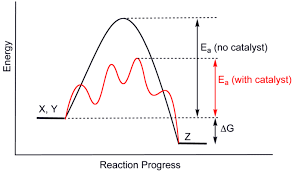The image above represents rate of heterogeneous reaction.

To compute for rate of heterogeneous reaction, two essential parameters are needed and these parameters are dn/dt and Amount of Material (n).

The formula for calculating rate of heterogeneous reaction:

V = dn/dt / n

Where:

V = Rate of Heterogeneous Reaction
dn/dt = dn/dt
n = Amount of Material

Let’s solve an example;
Find the rate of heterogeneous reaction when the dn/dt is 36 and the amount of material is 12.

This implies that;

dn/dt = dn/dt = 36
n = Amount of Material = 12

V = dn/dt / n
V = 24 / 12
V = 2

Therefore, the rate of heterogeneous reaction is 2.

Calculating the dn/dt when the Rate of Heterogeneous Reaction and the Amount of Material is Given.

dn/dt = V x n

Where;

dn/dt = dn/dt
V = Rate of Heterogeneous Reaction
n = Amount of Material

Let’s solve an example;
Find the dn/dt when the rate of heterogeneous reaction is 28 and the amount of material is 2.

This implies that;

V = Rate of Heterogeneous Reaction = 28
n = Amount of Material = 2

dn/dt = V x n
dn/dt = 28 x 2
dn/dt = 56

Therefore, the dn/dt is 56.

Calculating the Amount of Material when the Rate of Homogeneous Reaction and the dn/dt is Given.

n = dn/dt / V

Where;

n = Amount of Material
V = Rate of Heterogeneous Reaction
dn/dt = dn/dt

Let’s solve an example;
Find the amount of material when the rate of heterogeneous reaction is 12 and the dn/dt is 36.

This implies that;

V = Rate of Heterogeneous Reaction = 12
dn/dt = dn/dt = 36

n = dn/dt / V
n = 36 / 12
n = 3

Therefore, the amount of material is 3.

Nickzom Calculator – The Calculator Encyclopedia is capable of calculating the rate of heterogeneous reaction.

To get the answer and workings of the rate of heterogeneous reaction using the Nickzom Calculator – The Calculator Encyclopedia. First, you need to obtain the app.

You can get this app via any of these means:

To get access to the professional version via web, you need to register and subscribe for NGN 2,000 per annum to have utter access to all functionalities.
You can also try the demo version via https://www.nickzom.org/calculator

Apple (Paid) – https://itunes.apple.com/us/app/nickzom-calculator/id1331162702?mt=8
Once, you have obtained the calculator encyclopedia app, proceed to the Calculator Map, then click on Materials and Metallurgical under Engineering.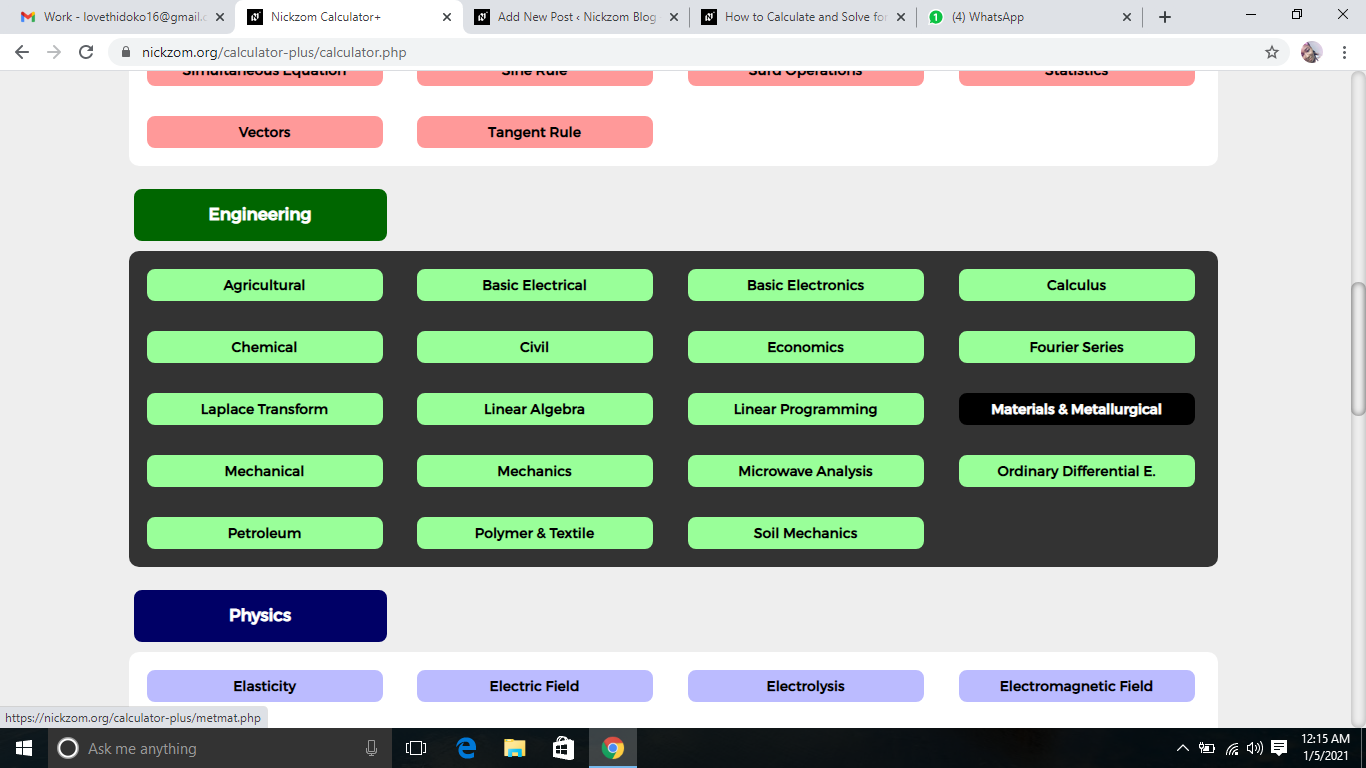Now, Click on Corrosion under Materials and Metallurgical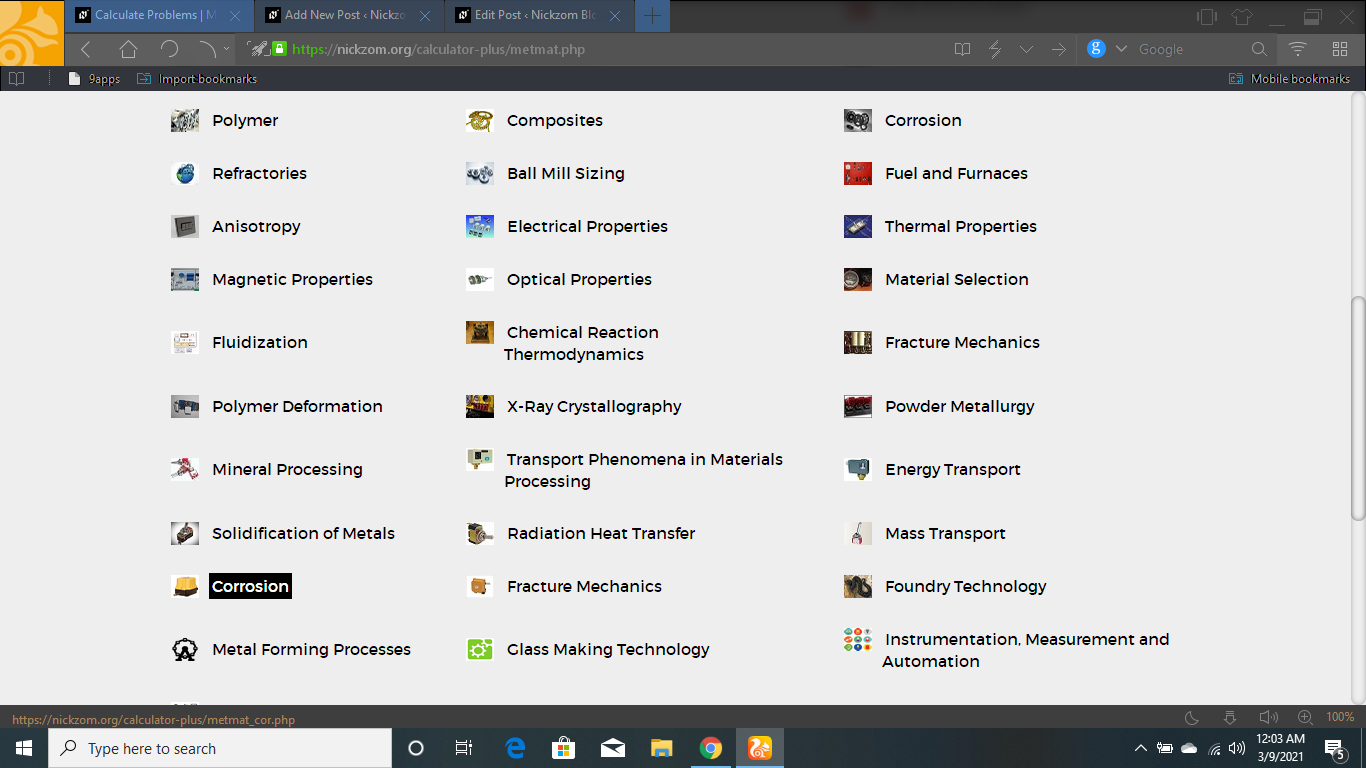Now, Click on Rate of Heterogeneous Reaction under Corrosion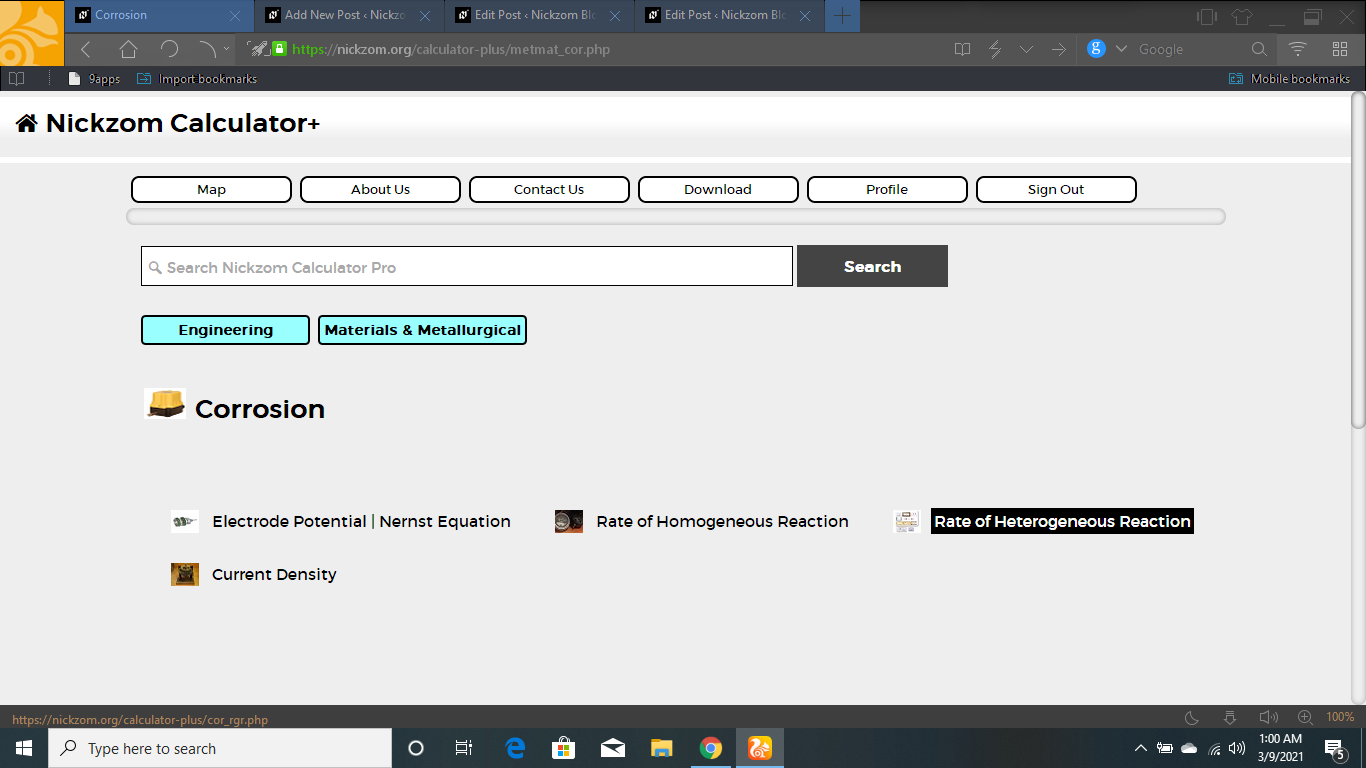The screenshot below displays the page or activity to enter your values, to get the answer for the rate of heterogeneous reaction according to the respective parameters which is the dn/dt and Amount of Material (n).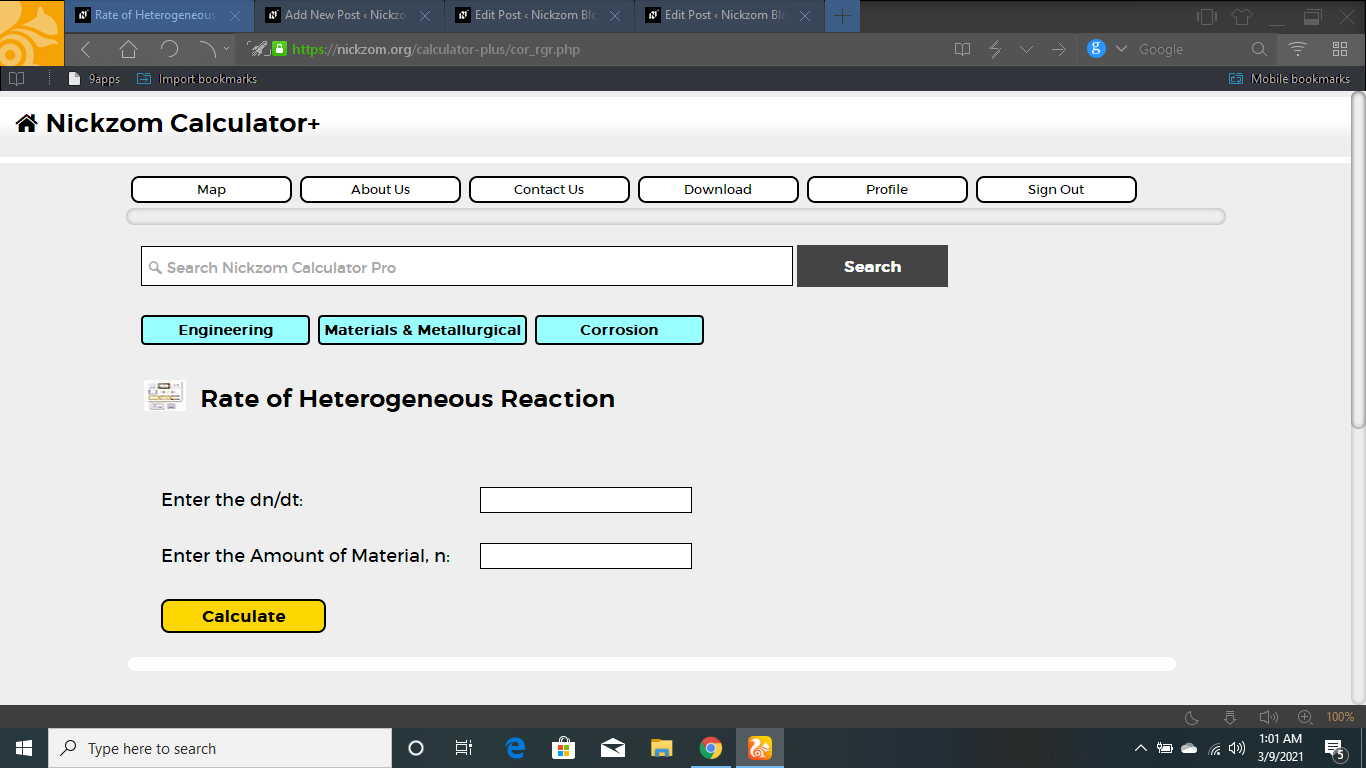Now, enter the value appropriately and accordingly for the parameter as required by the dn/dt is 36 and Amount of Material (n) is 12.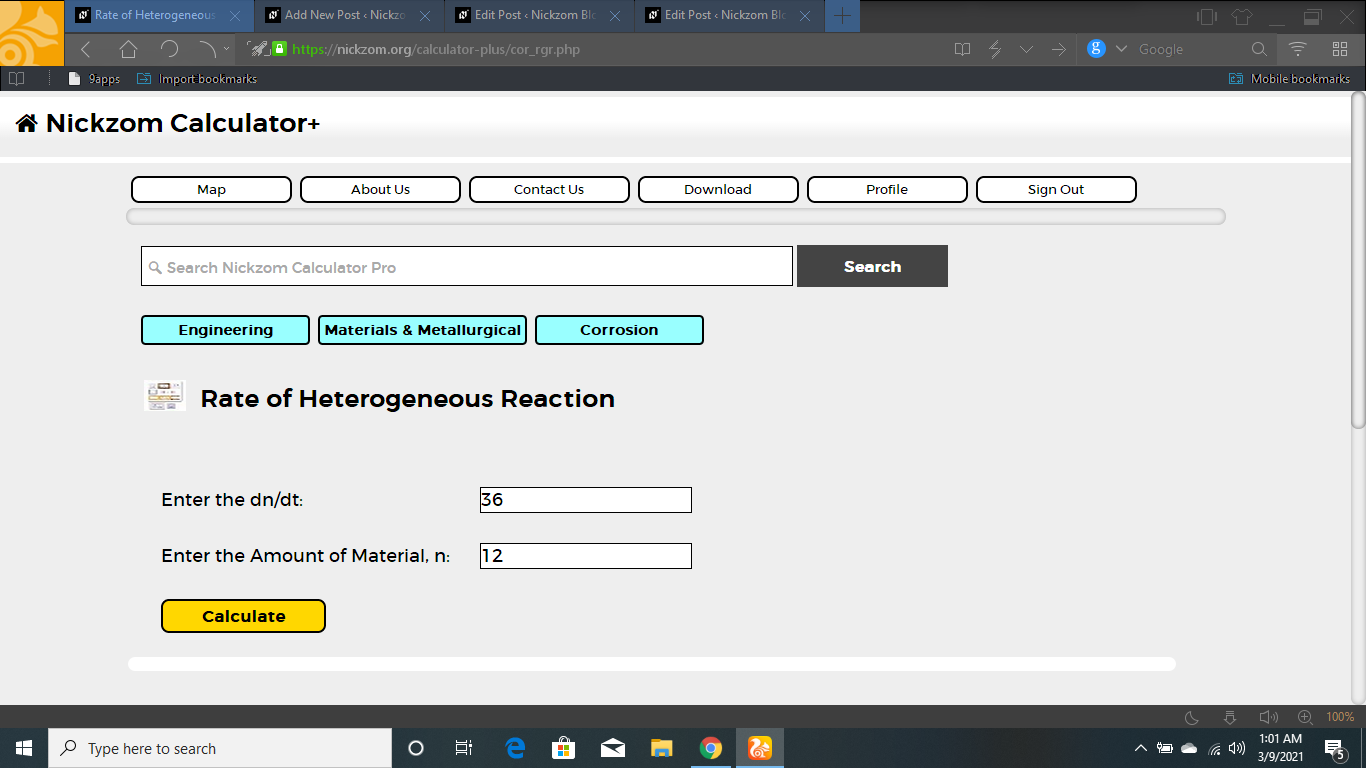Finally, Click on Calculate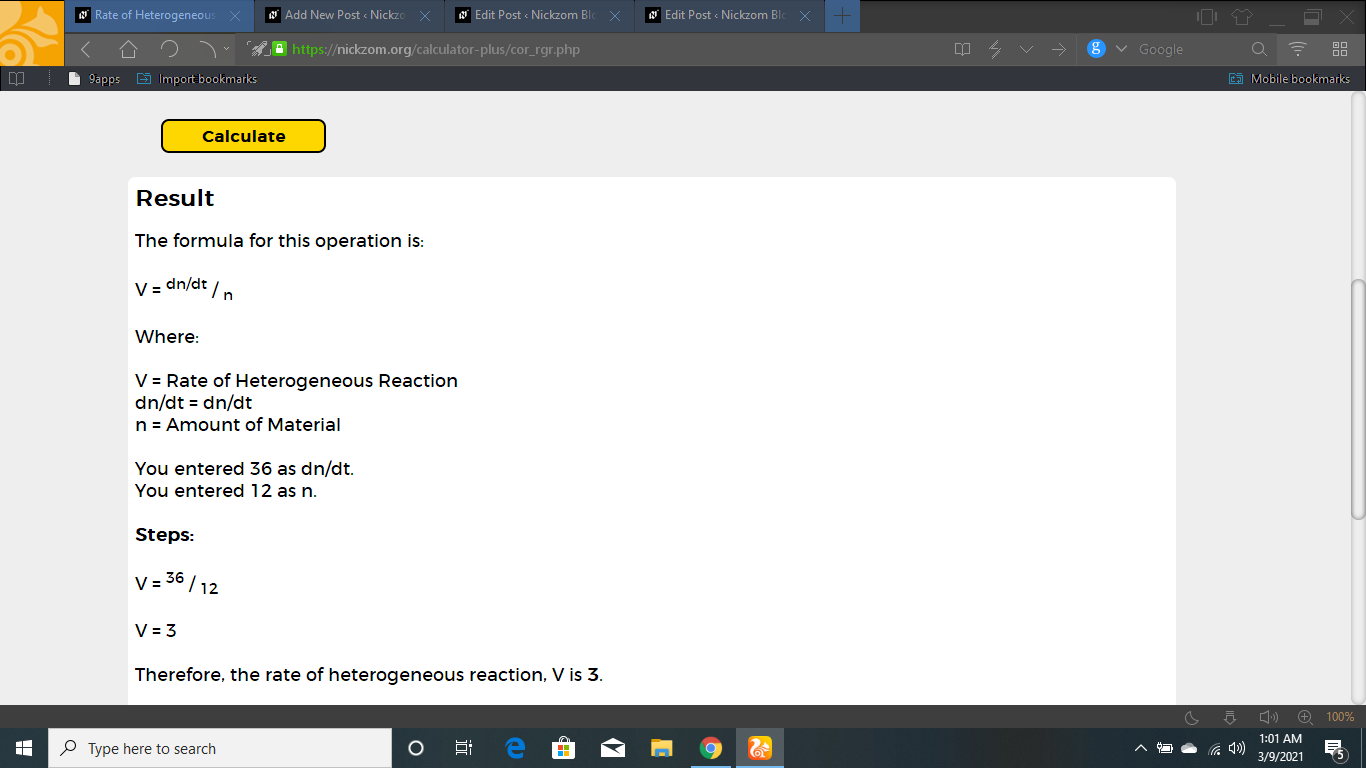As you can see from the screenshot above, Nickzom Calculator– The Calculator Encyclopedia solves for the rate of heterogeneous reaction and presents the formula, workings and steps too.